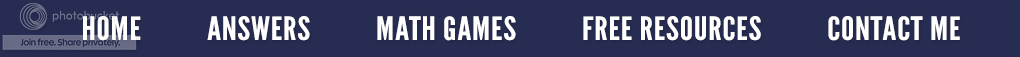### Problem Solving - Getting Unstuck!

Math courses are not like other courses. To pass most other subjects, a student must read, understand, and recall the subject matter. However, to pass math, an extra step is required: a student must use the information they have learned to solve math problems correctly. Special math study skills are needed to help the student learn more and to get better grades.

The study of mathematics should emphasize problem solving so that students can use and apply a wide variety of strategies to investigate and understand mathematical content. In this way, they acquire confidence in using mathematics meaningfully and the assurance that they can be successful in math.

But what is a real problem that requires problem solving? A real problem solving problem presents a challenge that cannot be resolved by some routine procedure known to the student and where the student accepts the challenge! Now, there's the dilemma, students who actually accept the challenge and are persistent enough to solve the problem.

What can we, as math instructors, do when students become frustrated, exasperated and discouraged and say they are stuck? Let's look at ten ways to help them get "unstuck".

Top Ten Ways to Get UnstuckFree Resource